## 线程、线程锁与线程安全的数据结构

2630 1 2018年11月28日

0 0 投票数

## 线程

### 线程的创建

#include <pthread.h>
int pthread_create(pthread_t* thread, const pthread_attr_t* attr, void* (*start_routine)(void*), void* arg);
• thread是一个线程的结构体，将它传递给pthread_create()来完成初始化。
• attr是一些必要的属性，例如设置堆栈大小、调度优先级。简单地传入NULL就可以使用默认的情况。
• start_routine是一个函数指针，表示了这个函数的名字，以及它的参数类型是void*，返回类型是一个void*。如果这个函数接受的是一个int参数，那么就可以写成：
int pthread_create( //...,
void* (*start_routine)(int), int arg);

或者，如果它返回值是一个int，那么就可以写成：

int pthread_create( //...,
int (*start_routine)(void*), void* arg);
• arg是传入线程开始执行的那个函数的参数，使用void*是为了可以传入任何类型的参数，以及返回任何类型的返回值。
#include <pthread.h>

typedef struct __myarg_t {
int a;
int b;
} myarg_t;

myarg_t *m = (myarg_t*) arg;
printf("%d %d\n", m->a, m->b);
return NULL;
}

int main(int argc, const char* argv[]){
int rc;
my_arg_t args;
args.a = 10;
args.b = 20;
//...
}

### 线程的结束

int pthread_join(pthread_t thread, void** value_ptr);

thread指定了要等待哪个线程执行结束。

value_ptr是指向那个希望得到的返回值的指针，由于返回值是一个void*，所以这里就是void**

#include <stdio.h>
#include <assert.h>
#include <stdlib.h>

typedef struct __myarg_t {
int a;
int b;

} myarg_t;

typedef struct __myret_t {
int x;
int y;
} myret_t;

myarg_t *m = (myarg_t*) arg;
printf("%d %d\n", m->a, m->b);
myret_t *r = Malloc(sizeof(myret_t));
r->x = 1;
r->y = 2;
return (void*) r;
}

int main(int argc, const char* argv[]){
myret_t *m;

myarg_t args = {10, 20};
printf("returned %d %d\n", m->x, m->y);
free(m);
return 0;
}

void* mythread(void* arg) {
myarg_t *m = (myarg_t*) arg;
printf("%d %d\n", m->a, m->b);
myret_t r; // ALLOCATED ON STACK: BAD!
r.x = 1;
r.y = 2;
return (void*) &r;
}

### 锁

int pthread_mutex_lock (pthread_mutex_t* mutex);
int pthread_mutex_unlock(pthread_mutex_t* mutex);

pthread_mutex_t lock;
x = x + 1; // or whatever your critical section is
pthread_mutex_unlock(&lock);

pthread_mutex_t lock = PTHREAD_MUTEX_INITIALIZER;
int rc = pthread_mutex_init(&lock, NULL);
assert(rc == 0); //always check success!

// Use this to keep your code clean but check for failures
// Only use if existing program is OK upon failure
assert(rc == 0);
}

int thread_mutex_trylock(pthread_mutex_t* mutex);

int thread_mutex_timedlock(pthread_mutex_t* mutex, struct timespec* abs_timeout);

### 条件变量

int pthread_cond_wait(pthread_cont_t* cond, pthread_mutex_t* mutex);
int pthread_cond_signal(pthread_cond_t* cond);

pthread_cond_wait()函数中，是将调用调用的线程进行睡眠，直到有别的线程去唤醒他。唤醒的情况，通常是现在程序中有些事情发生了改变，需要正在睡眠中的线程去注意这些改变。

pthread_mutex_t lock = PTHREAD_MUTEX_INITIALIZER;

Pthread_mutex_unlock(&lock);

Pthread_mutex_lock(&lock);
Pthread_mutex_unlock(&lock);

while (ready == 0)
; // spin
// 睡眠
ready = 1;
// 唤醒

### 一些建议

• 程序简单
• 线程交互尽量少
• 初始化锁和条件变量
• 检查返回代码
• 注意传入线程和线程返回的参数
• 每一个线程都有自己的栈
• 在线程之间唤醒的时候总是使用条件变量

## 锁

### 锁的基本思想

balance = balance + 1

lock_t mutex; // some globally-allocated lock 'mutex'
...
lock(&mutex);
balance = balance + 1;
unlock(&mutex);

pthread_mutex_t lock = PTHREAD_MUTEX_INITIALIZER;

balance = balance + 1;
Pthread_mutex_unlock(&lock);

• 一种粗粒度的做法是，使用一个大的锁来应对所有的不同的临界区的访问。
• 一种细粒度的做法是，不同的数据和数据结构各自都有各自的锁来保护，这样可以增加并发性，使得不同的线程可以同时访问不同的临界区。

### 评估一个锁

• 首先，要看锁是不是提供了互斥访问。
• 其次，锁是不是公平的。
• 也就是说，是不是所有渴望得到锁的线程都有平等的机会来获得锁，有没有可能锁只在某个或者某几个线程之间来回切换使得一部分线程永远处于饥饿的状态。
• 最后，性能也是重要的评估指标。

### 控制中断

void lock(){
DisableInterrupts();
}

void unlock(){
EnableInterrupts();
}

### 一个失败的尝试：只使用装载/存储

typedef struct __lock_t { int flag; } lock_t;

void init(lock_t * mutex) {
// 0 -> lock is avaliable, 1 -> held
mutex->flag = 0;
}

void lock(lock_t* mutex) {
while (mutex->flag == 1) // TEST the flag
; // spin-wait (do nothing)
mutex->flag = 1; //now SET it
}

void unlock(lock_t* mutex) {
mutex->flag = 0;
}### 使用 Test-And-Set 来构造一个自旋锁

int TestAndSet(int * old_ptr, int new){
int old = *old_ptr; // fetch old value at old_ptr
*old_ptr = new; // store 'new' into old_ptr
return old; // return the old value
}

int flag;
int turn;

void init(){
flag = flag = 0; // 1 -> thread wants to grab lock
turn = 0; // whose turn (thread 0 or 1)
}

void lock() {
flag[self] = 1; // self: threadID of caller
turn = 1 - self; // make it other thread's turn
while ((flag[1-self] == 1) && (turn == 1 - self))
; // spin-wait
}

void unlock() {
flag[self] = 0; // simply undo your intent
}

### Compare-And-Swap

int CompareAndSwap(int* ptr, int expected, int new) {
int actual = *ptr;
if (actual == expected)
*ptr = new;
return actual;
}

void lock(lock_t * lock){
while (CompareAndSwap(&lock->flag, 0, 1) == 1)
; //spin
}

char CompareAndSwap(int* ptr, int old, int new){
unsigned char ret;

// Note that sete sets a 'byte' not the word
__asm__ __volatile__ (
"  lock\n"
"  cmpxchg1 %2,%1\n"
"  sete %0\n"
: "=q" (ret), "=m" (*ptr)
: "r" (new), "m" (*ptr), "a" (old)
: "memory");
return ret;
}

### 链接加载和条件存储

int LoadLinked(int* ptr){
return *ptr;
}

int StoreConditional(int* ptr, int value){
*ptr = value;
return 1; // success!
} else {
return 0; // failed to update
}
}

LoadLinked()与普通的装载指令没有什么不同，就是把内存中的值装载到寄存器中。

void lock(lock_t* lock) {
while (1) {
; // spin until it's zero
if (StoreConditional(&lock->flag, 1) == 1)
return; // if set-it-to-1 was a success: all done
// otherwise: try it all over again
}
}

void unlock(loct_t* lock) {
lock->flag = 0;
}

void lock(lock_t* lock) {
; // spin
}

int FetchAndAdd(int * ptr){
int old = *pyr;
*ptr = old + 1;
return old;
}

typedef struct __lock_t {
int ticket;
int turn;
} lock_t;

void lock_init(lock_t* lock){
lock->ticket = 0;
lock->turn = 0;
}

void lock(lock_t* lock) {
while (lock->turn != myturn)
; // spin
}

void unlock(lock_t * lock){
lock->turn = lock->turn + 1;
}

### 睡眠

void int(){
flag = 0;
}

void lock(){
while (TestAndSet(&flag, 1) == 1){
yield(); // give up the CPU
}
}

void unlock(){
flag = 0;
}

### 睡眠队列

typedef struct __lock_t {
int flag;
int guard;
queue_t *q;
} lock_t;

void lock_init(lock_t* lock){
m->flag = 0;
m->guard = 0;
queue_init(m->q);
}

void lock(loct_t* m) {
while (TestAndSet(&m->guard, 1) == 1)
; // acquire guard lock by spinning
if (m->flag == 0) {
m->flag = 1; // lock is acquired
m->guard = 0;
} else {
m->guard = 0;
park();
}
}

void unlock(){
while (TestAndSet(&m->guard, 1) == 1)
; // acquired guard lock by spinning
if (queue_empty(m->q))
m->flag = 0; // let go of lock; no one wants it
else
unpark(queue_remove(m->q)); // hold lock (for next thread!)
m->guard = 0;
}

Solaris为了解决这个问题引入了一个新的函数setpark()

queue_add(m->q, gettid());
setpark(); // new code
m->guard = 0;

### 二相锁

Linux系统中有一种实现锁的旧方法，被称为二相锁。

void mutex_lock (int *mutex) {
int v;
/* Bit 31 was clear, we got the mutex (this is the fastpath) */
if (atomic_bit_test_set (mutex, 31) == 0)
return;
atomic_increment (mutex);
while (1) {
if (atomic_bit_test_set (mutex, 31) == 0) {
atomic_decrement (mutex);
return;
}
/* We have to wait now. First make sure the futex value
we are monitoring is truly negative (i.e. locked). */
v = *mutex;
if (v >= 0)
continue;
futex_wait (mutex, v);
}
}

void mutex_unlock (int *mutex) {
/* Adding 0x80000000 to the counter results in 0 if and only if
there are not other interested threads */
return;
/* There are other threads waiting for this mutex,
wake one of them up. */
futex_wake (mutex);
}

## 基于锁的并发数据结构

### 并发计数器

typedef struct __counter_t {
int value;
} count_t;

void init(counter_t* c){
c->value = 0;
}

void increment(counter_t* c){
c->value++;
}

void decrement(counter_t* c){
c->value--;
}

int get(counter_t* c){
return c->value;
}

typedef struct __counter_t {
int value;
} count_t;

void init(counter_t* c){
c->value = 0;
}

void increment(counter_t* c){
c->value++;
}

void decrement(counter_t* c){
c->value--;
}

int get(counter_t* c){
int rc = c->value;
return rc;
}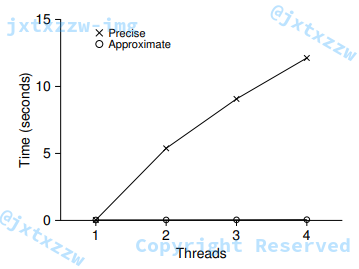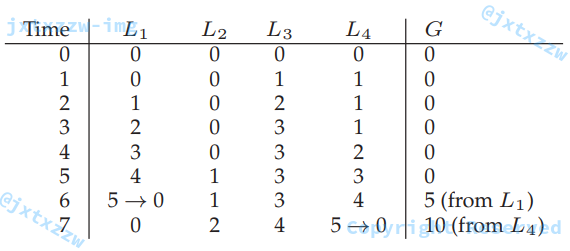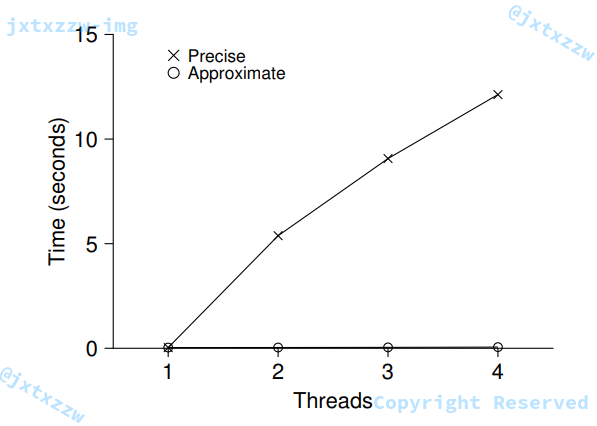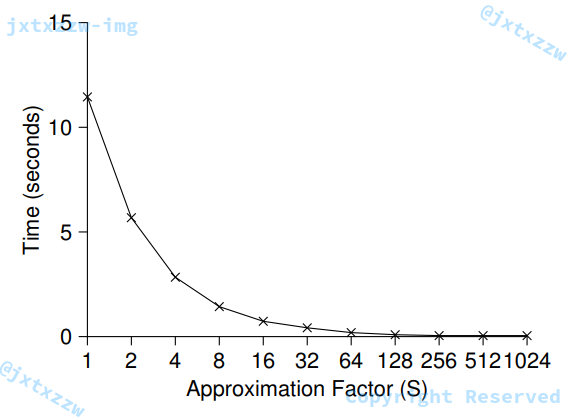typedef struct __counter_t {
int global; // global count
int local[NUMCPUS]; // local count (per cpu)
pthread_mutex_t llock[NUMCPUS]; // ... and locks
int threshold; // update frequency
} counter_t;

// init: record threshold, init locks, init values of all local counts and global count
void init(counter_t* c, int threshold) {
c->threshold = threshold;
c->global = 0;
int i;
for (i = 0; i < NUMCPUS; i++){
c->local[i] = 0;
}
}

// update: usually, just grab local lock and update local amount once local count has risen by 'threshold', grab global lock and transfer local values to it
void update(counter_t* c, int threadID, int amt){
int cpu = threadID % NUMCPUS;
c->local[cpu]  += amt; // assumes amt > 0
if (c->local[cpu] >= c->threshold) { // transfer to global
c->global += c->local[cpu];
c->local[cpu] = 0;
}
}

// get:just return global amount (which my not be perfect)
int get(counter_t* c) {
int val = c->global;
return val; // only approximate
}

int getAcuracy(counter_t* c) {
for (int i = 0; i < NUMCPUS; i++){
c->global += c->local[i];
c->local[i] = 0;
}
int val = c->global;
return val;
}

### 并发链表

// basic node structure
typedef struct __node_t {
int key;
struct __node_t* next;
} node _t;

// basic list structure (on used per list)
typedef struct __list_t {
} list_t;

void List_IInit(list_t* L){
}

int List_Insert(list_t* L, int key) {
node_t* new = malloc(sizeof(node_t));
if (new == NULL){
perror("malloc");
return -1; // fail
}
new->key = key;
return 0;
}

int List_Lookup(list_t* L, int key){
while (curr){
if (curr->key == key){
return 0; // success
}
curr = curr->next;
}
return -1;
}

malloc()本身就是线程安全的，每一个线程都可以调用它，而不需担心竞争条件或者其他并发的错误。

void List_Init(list_t* L){
}

void List_Insert(list_t *L, int key) {
// synchronization not needed
node_t *new = malloc(sizeof(node_t));
if (new == NULL) {
perror("malloc");
return;
}
new->key = key;

// just lock critical section
}

int List_Lookup(list_t *L, int key) {
int rv = -1;
while (curr) {
if (curr->key == key) {
rv = 0;
break;
}
curr = curr->next;
}
return rv; // now both success and failure
}

### 并发队列

typedef struct __node_t {
int value;
struct __node_t *next;
} node_t;

typedef struct __queue_t {
node_t *tail;
} queue_t;

void Queue_Init(queue_t *q) {
node_t *tmp = malloc(sizeof(node_t));
tmp->next = NULL;
}

void Queue_Enqueue(queue_t *q, int value) {
node_t *tmp = malloc(sizeof(node_t));
assert(tmp != NULL);
tmp->value = value;
tmp->next = NULL;

q->tail->next = tmp;
q->tail = tmp;
}

int Queue_Dequeue(queue_t *q, int *value) {
return -1; // queue was empty
}
free(tmp);
return 0;
}

### 并发哈希表

#define BUCKETS (101)

typedef struct __hash_t {
list_t lists[BUCKETS];
} hash_t;

void Hash_Init(hash_t *H) {
int i;
for (i = 0; i < BUCKETS; i++) {
List_Init(&H->lists[i]);
}
}

int Hash_Insert(hash_t *H, int key) {
int bucket = key % BUCKETS;
return List_Insert(&H->lists[bucket], key);
}

int Hash_Lookup(hash_t *H, int key) {
int bucket = key % BUCKETS;
return List_Lookup(&H->lists[bucket], key);
}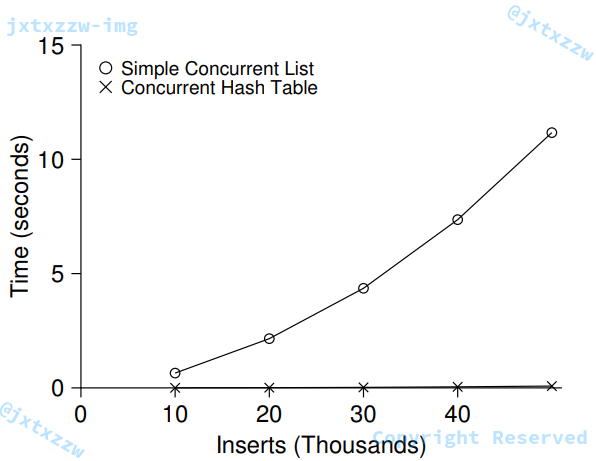0 0 投票数

1条留言（可选）如果您也有个人网站，不妨分享一下

1 评论sscjwdyzdw(@sscjwdyzdw)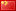Chrome 67.0.3396.99 Windows 10
2018年12月5日 20:35

HHH

Warning: error_log(/home/wwwroot/www.jxtxzzw.com/wp-content/plugins/spider-analyser/#log/log-1013.txt): failed to open stream: No such file or directory in /home/wwwroot/www.jxtxzzw.com/wp-content/plugins/spider-analyser/spider.class.php on line 2900